# Applications of PGMs and relation and conversion of Bayes and Markov's model

In this article, we will know more about PGMs: its application, Relation between Bayes network and Markov's Random field and conversion of Bayes network into Markov's random field.
Submitted by Bharti Parmar, on March 16, 2019

## Application of PGMs: (Use cases)

• Netflix, Amazon, facebook all use PGM recommend what is best for you.
• This algorithm, Use the latent factor model and non-negative matrix factorization.
• In politics American presidential polls using PGMs for prediction. FiveThirtyEight company that makes a prediction about American presidential polls using PGMs.
• PGM is also inferred whether one is a MODI and KEJRIWAL supporter.

How Bayes network and Markov Random Fields Model related to each other: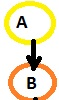For P(A, B) = P(A) * P(B/A) For P(A,B) α Ф(A,B)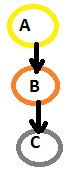For chain: P(A,B) = P(A) P(B/A) P(C/B) For chain: P(A,B) α Ф(A,B) Ф(B,C)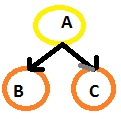Shared parents: P(A,B,C) = P(A) P(B/A) P(C/B) Shared parents: P(A,B,C) α Ф(A,B) Ф(A,C)Ф(A,B) ← P(A) P(B\A)Ф(A,C) ← P(C\A)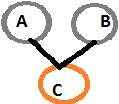Two parent shared a child: P(A,B,C) = P(A) P(B) P(C\A,B) A and B are independent given C P(A,B,C) α Ф(A,C) Ф(B,C)

Converting Bayes network into Markov random fields: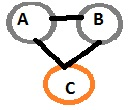Moralizing parents: P (A, B, C) α Ф(A,C) Ф(B,C) where A and B are independent given C

• Moralize all co-parents
• More challenging is that Lose marginal independence of parents.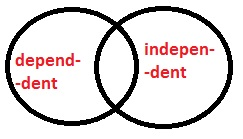Basically, it given an interference class of all directed graphical models and a class of all undirected graphical model are different but overlap each other.

Conclusion: In this article we have learnt about application of PGMs, how bayes network is related to markov's random field and conversion of bayes network into Markov's network model.

What's New

Top Interview Coding Problems/Challenges!

Languages: » C » C++ » C++ STL » Java » Data Structure » C#.Net » Android » Kotlin » SQL
Web Technologies: » PHP » Python » JavaScript » CSS » Ajax » Node.js » Web programming/HTML
Solved programs: » C » C++ » DS » Java » C#
Aptitude que. & ans.: » C » C++ » Java » DBMS
Interview que. & ans.: » C » Embedded C » Java » SEO » HR
CS Subjects: » CS Basics » O.S. » Networks » DBMS » Embedded Systems » Cloud Computing
» Machine learning » CS Organizations » Linux » DOS
More: » Articles » Puzzles » News/Updates